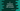# C toUpper() method explanation with example## C toUpper() method :

toUpper is defined in Ctype.h header file. It is used to convert one lowercase letter to the uppercase. If the conversion is not possible, it returns the same letter. As it is defined in Ctype.h, we need to import this header in our program.

### Syntax of toUpper() :

The syntax of toUpper is as below :

``````int toUpper(int i)
``````

You can see that the parameter is of integer type and also it returns one integer. But we can pass one character. It will typecast the character to integer i.e. to its ASCII value. It will then return the ASCII value of the uppercase character. Let me show you one example of how it works :

### C program Example of toUpper() :

Consider the below example :

``````#include<stdio.h>
#include<ctype.h>

int main(){
printf("%d\n",toupper('a'));
printf("%c\n",toupper('a'));
}``````

We have two print statements here. Both statements passes ‘a’ to toupper. If you run this program, it will print the below output :

``````65
A
``````

The first printf prints the ASCII value of ‘A’ i.e. 65. This value is returned by this method. But the second printf prints ‘A’ by converting 65 to ‘A’. Note that we are using the ctype.h header file to use this method.

### C program to convert all characters of a string to upper case :

We can also use this method to convert all characters of a string to the upper case like below :

``````#include <stdio.h>
#include <ctype.h>

int main()
{
char inputStr[] = "hello World !!";
char outputStr;
int i = 0;

while (inputStr[i])
{
outputStr[i] = toupper(inputStr[i]);
i++;
}

printf("Given string : %s\n", inputStr);
printf("Converted string : %s\n", outputStr);
}``````

### Explanation :

• In this program, inputStr is the given string or character array. We are converting all characters of this string to uppercase.

• The final string is stored in outputStr character array.

• We have initialized one variable i as 0. This variable is used to iterate through the characters of the string.

• Using the while loop, we are iterating through the characters, converting each character to uppercase and inserting them to the array outputStr. We have uppercase character and special characters in the input string. These characters are not converted and remains the same.

It will print the below output :

``````Given string : hello World !!
Converted string : HELLO WORLD !!
``````